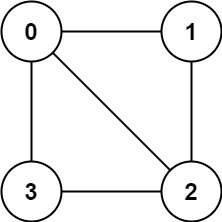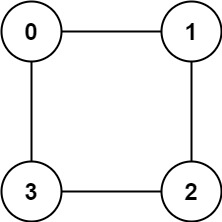## Algorithm

Problem Name: 785. Is Graph Bipartite?

There is an undirected graph with `n` nodes, where each node is numbered between `0` and `n - 1`. You are given a 2D array `graph`, where `graph[u]` is an array of nodes that node `u` is adjacent to. More formally, for each `v` in `graph[u]`, there is an undirected edge between node `u` and node `v`. The graph has the following properties:

• There are no self-edges (`graph[u]` does not contain `u`).
• There are no parallel edges (`graph[u]` does not contain duplicate values).
• If `v` is in `graph[u]`, then `u` is in `graph[v]` (the graph is undirected).
• The graph may not be connected, meaning there may be two nodes `u` and `v` such that there is no path between them.

A graph is bipartite if the nodes can be partitioned into two independent sets `A` and `B` such that every edge in the graph connects a node in set `A` and a node in set `B`.

Return `true` if and only if it is bipartite.

Example 1:```Input: graph = [[1,2,3],[0,2],[0,1,3],[0,2]]
Output: false
Explanation: There is no way to partition the nodes into two independent sets such that every edge connects a node in one and a node in the other.```

Example 2:```Input: graph = [[1,3],[0,2],[1,3],[0,2]]
Output: true
Explanation: We can partition the nodes into two sets: {0, 2} and {1, 3}.```

Constraints:

• `graph.length == n`
• `1 <= n <= 100`
• `0 <= graph[u].length < n`
• `0 <= graph[u][i] <= n - 1`
• `graph[u]` does not contain `u`.
• All the values of `graph[u]` are unique.
• If `graph[u]` contains `v`, then `graph[v]` contains `u`.

## Code Examples

### #1 Code Example with C++ Programming

```Code - C++ Programming```

``````
class Solution {
public:
bool isBipartite(vector>& graph) {
int n = graph.size();
vectorcolor(n);
color = 1;
for(int i = 0; i < n; i++){
auto neigh = graph[i];
if(!color[i]) for(auto j: neigh) if(color[j]){ color[i] = -color[j]; break; }   // If un-colored, pick a color by neighbor
if(!color[i]) color[i] = 1;  // Empty neighbor or no colored neighbor, colored 1 as default
for(auto j: neigh)
if(!color[j]) color[j] = -color[i];
else if(color[i] != -color[j]) return false;
}
return true;
}
};
``````
Copy The Code &

Input

cmd
graph = [[1,2,3],[0,2],[0,1,3],[0,2]]

Output

cmd
false

### #2 Code Example with Java Programming

```Code - Java Programming```

``````
class Solution {
public boolean isBipartite(int[][] graph) {
int n = graph.length;
int[] color = new int[n];
Arrays.fill(color, -1);
for (int i = 0; i < n; i++) {
if (color[i] == -1 && !bipartiteCheck(graph, i, color)) {
return false;
}
}
return true;
}

private boolean bipartiteCheck(int[][] graph, int node, int[] color) {
int currColor = 0;
while (!queue.isEmpty()) {
int size = queue.size();
while (size-- > 0) {
int removed = queue.remove();
if (color[removed] != -1) {
if (color[removed] != currColor) {
return false;
}
continue;
}
color[removed] = currColor;
for (int conn : graph[removed]) {
if (color[conn] == -1) {
}
}
}
currColor = currColor == 1 ? 0 : 1;
}
return true;
}
}
``````
Copy The Code &

Input

cmd
graph = [[1,2,3],[0,2],[0,1,3],[0,2]]

Output

cmd
false

### #3 Code Example with Javascript Programming

```Code - Javascript Programming```

``````
const isBipartite = function (graph) {
const visited = Array(graph.length).fill(0);
for (let i = 0; i < graph.length; i++) {
if (visited[i] !== 0) {
continue;
}
const queue = [];
queue.push(i);
visited[i] = 1;
while (queue.length > 0) {
const index = queue.shift();
for (let j = 0; j < graph[index].length; j++) {
const temp = graph[index][j];
if (visited[temp] === 0) {
visited[temp] = visited[index] * -1;
queue.push(temp);
} else {
if (visited[temp] === visited[index]) return false;
}
}
}
}
return true;
};
``````
Copy The Code &

Input

cmd
graph = [[1,3],[0,2],[1,3],[0,2]]

Output

cmd
true

### #4 Code Example with Python Programming

```Code - Python Programming```

``````
class Solution:
def isBipartite(self, graph):
side =  * len(graph)
def dfs(node):
for v in graph[node]:
if side[v] == 0:
side[v] = -side[node]
if not dfs(v): return False
elif side[v] == side[node]: return False
return True
for i in range(len(graph)):
if side[i] == 0:
side[i] = 1
if not dfs(i): return False
return True
``````
Copy The Code &

Input

cmd
graph = [[1,3],[0,2],[1,3],[0,2]]

Output

cmd
true

### #5 Code Example with C# Programming

```Code - C# Programming```

``start coding...``
Copy The Code &